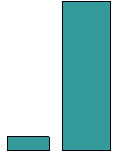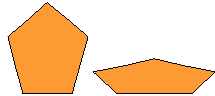Email us to get an instant 20% discount on highly effective K-12 Math & English kwizNET Programs!

#### Online Quiz (WorksheetABCD)

Questions Per Quiz = 2 4 6 8 10

### MEAP Preparation - Grade 7 Mathematics1.145 Geometry Part Three - Review Test

 Q 1: In the figure shown are the two figures similar?NoYes Q 2: The ____ of a figure is how much space it occupies.areaperimetervolume Q 3: What is the area of a rectangle whose length is 5 cm and width is 3 cm16 sq cm8 sq cm10 sq cm15 sq cm Q 4: Length of a rectangle is 12 cm and its total area is 84 square cm. Find its width. (hint: 12 cm x __ = 84 sq cm)8 cm12 cm7 cm Q 5: What is the volume of a cube with length 3 cm27 cubic cm18 cubic cm9 cubic cm Q 6: A triangular prism has ______ edges.6394 Q 7: Are the two triangles in the figure congruent? (hint: sometimes you have to turn the figure around to see if it is congruent with another)noyes Q 8: Are these similar figures?YesNo Question 9: This question is available to subscribers only! Question 10: This question is available to subscribers only!